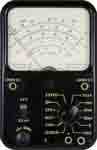# What is an Analog Multimeter - Analogue Meter?

### - an overview or tutorial about what an analogue or analog multimeter is, how to use it and how it works.

Multimeter Tutorial Includes:
Test meter basics     Analogue multimeter     DMM digital multimeter     How a DMM works     DMM accuracy & resolution     How to buy best digital multimeter     How to use a multimeter     Voltage measurement     Current measurements     Resistance measurements     Diode & transistor test     Fault finding transistor circuits

A analog or analogue multimeter is one of the trusty workhorses of the electronics test industry. Analogue multimeters have been in use for very many years and sometimes go by the name VOA as a result of the fact that they measure volts, ohms and amps. These multimeters are extremely flexible and enable very many faults to be found in an electronic circuit.

### ... analogue multimeters have been available for many years and they are very flexible in their operation....## Analog multimeter ranges

Analog multimeters, like digital ones have a variety of ranges. They are described in terms of Full Scale Deflection or FSD. This is the maximum that the range can read. In order to get the best reading, it is necessary to have the scale reading somewhere between about a quarter and all of the FSD. In this way the optimum accuracy and significant number of figures can be read. As a result of this meters have a variety of ranges, that may appear to be reasonably close to each other.

A typical meter may have the following ranges (note that the figures indicate the FSD):

• DC Voltage: 2.5V, 10V, 25V, 100V, 250V, 1000V
• AC voltage: 10V, 25V, 100V, 250V, 1000V
• DC Current: 50µA, 1mA 10mW, 100mA
• Resistance: R, 100R, 10 000R

There are several points to note from this typical analogue multimeter specification:

1. The low voltage AC voltage, and in this example the 10V AC range may have a different scale to the others. The reason for this is that at low voltages a bridge rectifier is non-linear and this needs to be taken into consideration. It is also for this reason that no 2.5V AC range was included.
2. The 1000V or 1kV ranges will often use a different input connection to enable the reading to be taken through a different shunt and kept away from the rotary switch that may not be able to handle a voltage this high.
3. AC current is often not included in the lower end meters because of the difficulties of undertaking the measurement without a transformer to step up any voltage across a series sensing resistor for rectification.
4. Batteries inside the multimeter are used to provide a current for the resistance measurements. No other readings require the use of battery power - the meter is passive from that viewpoint.
5. The three resistance ranges of varying sensitivity multiply the meter reading by 1, 100, or 10 000 dependent upon the range. This allows for low resistance measurements to be made as well as very high ones. Typically the higher resistance ranges may use a higher voltage battery than the one used for the low resistance ranges.

## Analog multimeter sensitivity

One of the specifications for an analogue multimeter is its sensitivity. This comes about because the meter must draw a certain amount of current from the circuit it is measuring in order for the meter to deflect. Accordingly the meter appears as another resistor placed between the points being measured. The way this is specified is in terms of a certain number of Ohms (or more usually kOhms) per volt. The figure enables the effective resistance to be calculated for any given range.

Thus if a multimeter had a sensitivity of 20 kOhms per volt, then on the range having a full scale deflection of 10 volts, it would appear as a resistance of 10 x 20 kohms, i.e. 200 kohms.

When making measurements the resistance of the meter should be at the very least ten times the resistance of the circuit being measured. As a rough guide, this can be taken to be the highest resistor value near where the meter is connected.

Normally the sensitivity of an analog meter is much less on AC than DC. A meter with a DC sensitivity of 20 kohms per volt on DC might only have a sensitivity of 1 kohm per volt on AC.